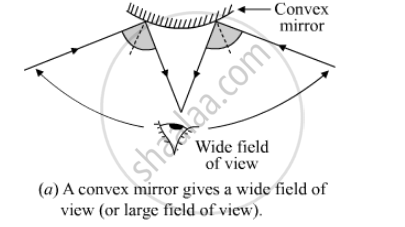Share

# Draw Diagram to Show How a Convex Mirror Can Be Used to Give a Large Field of View. - CBSE Class 10 - Science

ConceptReflection of Light Image Formation by Spherical Mirrors - Convex Mirror

#### Question

Draw diagram to show how a convex mirror can be used to give a large field of view.

#### Solution

The following ray diagram shows that a convex mirror can be used to produce a large field of view. This is because when an object is in front of the convex mirror, irrespective of its distance (other than infinity), a virtual, erect and diminished image of the former is obtained. So, a convex mirror can produce image of a large number of objects at the same time.Is there an error in this question or solution?

#### Video TutorialsVIEW ALL 

Solution Draw Diagram to Show How a Convex Mirror Can Be Used to Give a Large Field of View. Concept: Reflection of Light - Image Formation by Spherical Mirrors - Convex Mirror.
S